## Overview

This guide shows how Neon technology has been used to improve performance in the real-world: specifically, in the open-source Chromium project.

In the guide, we demonstrate to programmers who are unfamiliar with Neon how they can use intrinsics in their code to enable Single Instruction, Multiple Data (SIMD) processing. Using Neon in this way can bring huge performance benefits.

### What are Neon intrinsics?

Neon technology provides a dedicated extension to the Arm Instruction Set Architecture, providing additional instructions that can perform mathematical operations in parallel on multiple data streams.

Neon technology can help speed up a wide variety of applications, including:

• Audio and video processing
• 2D and 3D gaming graphics
• Voice and facial recognition
• Computer vision and deep learning

Neon intrinsics are function calls that programmers can use in their C or C++ code. The compiler then replaces these function calls with an appropriate Neon instruction or sequence of Neon instructions.

Intrinsics provide almost as much control as writing assembly language, but leave low-level details such as register allocation and instruction scheduling to the compiler. This frees developers to concentrate on the higher-level behavior of their algorithms, rather than the lower-level implementation details.

Another advantage of using intrinsics is that the same source code can be compiled for different targets. This means that, for example, a single source code implementation can be built for both 32-bit and 64-bit targets.

### Why Chromium?

Why did we choose Chromium to investigate the performance improvements possible with Neon?

Chromium provides the basis for Google Chrome, which is the most popular web browser in the world, in terms of user numbers. Any performance improvements that we made to the Chromium codebase can benefit many millions of users worldwide.

Chromium is an open-source project, so everyone can inspect the full source code. When learning about a new subject, like programming with Neon intrinsics, it often helps to have examples to learn from. We hope that the examples that are provided in this guide will help, because you can see them in the context of a complete, real-world codebase.

### Why PNG?

Now that we have decided to work in Chromium, where should we look in the Chromium code to make optimizations? With over 25 million lines of code, we must pick a specific area to target. When looking at the type of workloads that web browsers deal with, the bulk of content is still text and graphics. Images often represent most of the downloaded bytes on a web page, and contribute to a significant proportion of the processing time. Recent data suggests that 53% of mobile users abandon sites that take over 3 seconds to load. This means that optimizing image load times, and therefore page load times, should bring tangible benefits.

The Portable Network Graphics (PNG) format was developed as an improved, non-patented replacement for the Graphics Interchange Format (GIF). PNG is the standard for transparent images in the web. It is also a popular format for web graphics in general. Because of this, Arm decided to investigate opportunities for Neon optimization in PNG image processing.

### Introducing Bobby the bird

To help decide where to look for optimization opportunities, we went in search of performance data.

The following image of a bird has complex textures, a reasonably large size, and a transparent background. This means that it is a good test case for investigating optimizations to the PNG decoding process: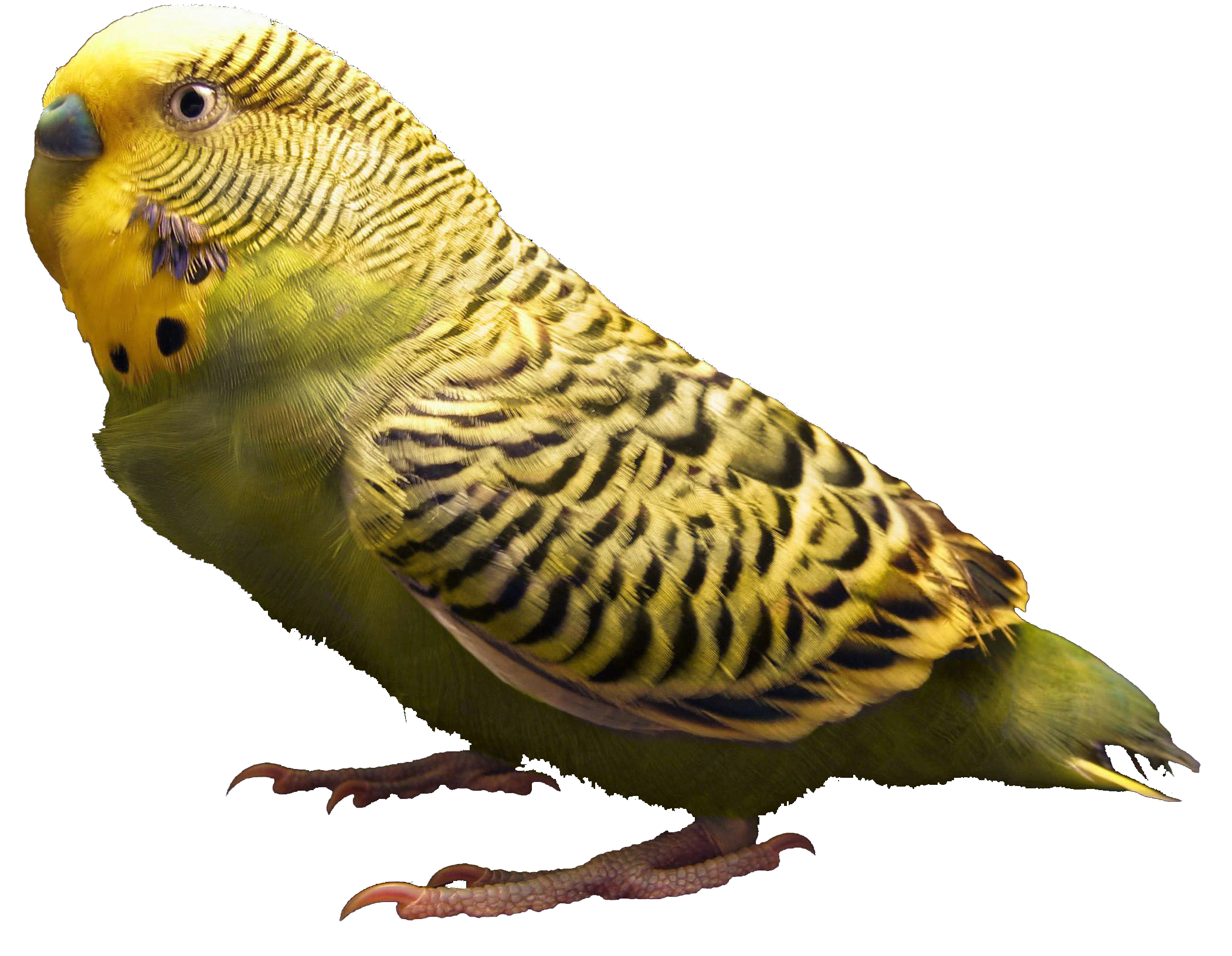Image source: Penubag [Public domain], via Wikimedia Commons

The first thing to know is that all PNG images are not created equally. There are several different ways to encode PNG images, for example:

• Compression. Different compression algorithms can result in different file sizes. For example, Zopfli produces PNG image files that are typically about 5% smaller than zlib, at the cost of taking longer to perform the compression.
• Pre-compression filters. The PNG format allows filtering of the image data to improve compression results. PNG filters are lossless, so they do not affect the content of the image itself. Filters only change the data representation of the image to make it more compressible. Using pre-compression filters can give smaller file sizes at the cost of increased processing time.
• Color depth. Reducing the number of colors in an image reduces file size, but also potentially degrades image quality.
• Color indexing. The PNG format allows individual pixel colors to be specified as either a TrueColor RGB triple, or an index into a palette of colors. Indexing colors reduces file sizes, but may degrade image quality if the original image contains more colors than the maximum that the palette allows. Indexed colors also need decoding back to the RGB triple, which may increase processing time.

We investigated performance with three different versions of the Bobby the budgie image to investigate possible areas for optimization.

Image File size Number of colors Palette or TrueColor? Filters? Compression Encoder
`Original_Bobby.PNG` 2.7M 211787 TrueColor Yes zlib libpng
`Palette_Bobby.PNG` 0.9M 256 Palette No zlib libpng
`Zopfli_Bobby.PNG` 2.6M 211787 TrueColor Yes Zopfli ZopfliPNG

To obtain performance data for each of these three images, we used the Linux perf tool to profile ContentShell. The performance data for each image is as follows:

```Original_Bobby.PNG
== Image has pre-compression filters (2.7MB) ==
Lib	 	Command	SharedObj			 method				CPU (%)
```Palette_Bobby.PNG
== Image has no pre-compression filters (0.9MB) ==
Lib	 	Command	SharedObj			 method				CPU (%)
```Zopfli_Bobby.PNG
== Image was optimized using zopfli (2.6MB) ==
Lib	 	Command	SharedObj			 method				CPU (%)

This data helped identify the zlib library as a good target for our optimization efforts. This is because it contains several methods that contribute significantly to performance.

Zlib was also considered a good candidate to target for the following reasons:

• The zlib library is used in many different software applications and libraries, for example libpng, Skia, FreeType, Cronet, and Chrome. This means that any performance improvements that we could achieve in zlib would yield performance improvements for many users.
• Released in 1995, the zlib library has a relatively old codebase. Older codebases might have areas that have not been modified in many years. These areas are likely to provide more opportunities for improvement.
• The zlib library did not contain any existing optimizations for Arm. This means that there probably a wide range of improvements to make.

Adler-32 is a checksum algorithm used by the zlib compression library to detect data corruption errors. Adler-32 checksums are faster to calculate than CRC32 checksums, but trade reliability for speed as Adler-32 is more prone to collisions.

The PNG format uses Adler-32 for uncompressed data and CRC32 is used for the compressed segments.

An Adler-32 checksum is calculated as follows:

• A is a 16-bit checksum calculated as the sum of all bits in the input stream, plus 1, modulo 65521.
• B is a 16-bit checksum calculated as the sum of all individual A values, modulo 65521. B has the initial value 0.
• The final Adler-32 checksum is a 32-bit checksum formed by concatenating the two 16-bit values of A and B, with B occupying the most significant bytes.

This means that the Adler-32 checksum function can be expressed as follows:

```A = 1 + D1 + D2 + ... + Dn (mod 65521)

B = (1 + D1) + (1 + D1 + D2) + ... + (1 + D1 + D2 + ... + Dn) (mod 65521)
= nxD1 + (n-1) x D2 + (n-2) x D3 + ... + Dn + n (mod 65521)

Adler-32(D) = (B x 65536) + A```

For example, the following table shows the calculation of the Adler-32 checksum of the ASCII string Neon:

Character Decimal ASCII code A B
N 78 (1 + 78) % 65521 = 79 (0 + 79) % 65521 = 79
e 101 (79 + 101) % 65521 = 180 (79 + 180) % 65521 = 259
o 111 (180 + 111) % 65521 = 291 (259 + 291) % 65521 = 550
n 110 (291 + 110) % 65521 = 401 (550 + 401) % 65521 = 951

The decimal Adler-32 checksum is calculated as follows:

```Adler-32 = (B x 65536) + A
= (951 x 65536) + 401
= 62,324,736 + 401
= 62,325,137```

The same calculation in hexadecimal is as follows:

```Adler-32 = (B x 00010000) + A
= (03B7 x 00010000) + 0191
= 03B70000 + 0191
= 03B70191```

### Unoptimized implementation

The following code shows a simplistic implementation of the Adler-32 algorithm, from Wikipedia:

```const uint32_t MOD_ADLER = 65521;

uint32_t adler32(unsigned char *data, size_t len)
/*
where data is the location of the data in physical memory and
len is the length of the data in bytes
*/
{
uint32_t a = 1, b = 0;
size_t index;

// Process each byte of the data in order
for (index = 0; index < len; ++index)
{
a = (a + data[index]) % MOD_ADLER;
b = (b + a) % MOD_ADLER;
}

return (b << 16) | a;
}```

This code simply loops through the data one value at a time, summing and accumulating results. One of the problems with this approach is that performing the modulo operation is expensive. Here, this expensive modulo operation is performed at every single iteration.

### Neon-optimized implementation

Optimizing the Adler-32 algorithm with Neon uses vector multiplication and accumulation to process up to 32 data values at the same time:

```static void NEON_accum32(uint32_t *s, const unsigned char *buf,
z_size_t len)
{
/* Please refer to the 'Algorithm' section of:
* Here, 'taps' represents the 'n' scalar multiplier of 'B', which
* will be multiplied and accumulated.
*/
static const uint8_t taps = {
32, 31, 30, 29, 28, 27, 26, 25,
24, 23, 22, 21, 20, 19, 18, 17,
16, 15, 14, 13, 12, 11, 10, 9,
8, 7, 6, 5, 4, 3, 2, 1 };

/* This may result in some register spilling (and 4 unnecessary VMOVs). */
const uint8x16_t t0 = vld1q_u8(taps);
const uint8x16_t t1 = vld1q_u8(taps + 16);
const uint8x8_t n_first_low = vget_low_u8(t0);
const uint8x8_t n_first_high = vget_high_u8(t0);
const uint8x8_t n_second_low = vget_low_u8(t1);
const uint8x8_t n_second_high = vget_high_u8(t1);

uint8x16_t d0, d1;

uint32x4_t s2acc = vdupq_n_u32(0);
s2acc = vsetq_lane_u32(s, s2acc, 0);

/*  Think of it as a vectorized form of the code implemented to
* handle the tail (or a DO16 on steroids). But in this case
* we handle 32 elements and better exploit the pipeline.
*/
while (len >= 2) {
d0 = vld1q_u8(buf);
d1 = vld1q_u8(buf + 16);
sum2 = vmull_u8(n_first_low, vget_low_u8(d0));
sum2 = vmlal_u8(sum2, n_first_high, vget_high_u8(d0));
sum2 = vmlal_u8(sum2, n_second_low, vget_low_u8(d1));
sum2 = vmlal_u8(sum2, n_second_high, vget_high_u8(d1));
len -= 2;
buf += 32;
}

/* This is the same as before, but we only handle 16 elements as
* we are almost done.
*/
while (len > 0) {
d0 = vld1q_u8(buf);
sum2 = vmull_u8(n_second_low, vget_low_u8(d0));
sum2 = vmlal_u8(sum2, n_second_high, vget_high_u8(d0));
buf += 16;
len--;
}

/* Combine the accumulated components (adler and sum2). */

/* Store the results. */
s = vget_lane_u32(as, 0);
s = vget_lane_u32(as, 1);
}```

The taps optimization that is referred to in the code comments works by computing the checksum of a vector of 32 elements where the n variable is known and fixed. This computed checksum is later recombined with another segment of 32 elements, rolling through the input data array. For more information, you can watch the BlinkOn 9: Optimizing image decoding on Arm presentation.

Elsewhere in the code, the expensive modulo operation is optimized so that it is only run when absolutely needed. The point at which the modulo is needed is just before the accumulated sum could possibly overflow the modulo value. This is calculated to be once every 5552 iterations.

Intrinsic Description
`vaddq_u32` Vector add.
`vdupq_n_u32` Load all lanes of vector to the same literal value.
`vget_high_u32`
`vget_high_u8`
`vget_low_u32`
`vget_low_u8`
Split vectors into two components.
`vget_lane_u32` Extract a single lane from a vector.
`vld1q_u8` Load a single vector or lane.
`vmlal_u8` Vector multiply and accumulate.
`vmull_u8` Vector multiply.
`vpadalq_u16`
`vpadalq_u8`
`vpadd_u32`
`vpaddlq_u8`
`vsetq_lane_u32` Load a single lane of a vector from a literal.
`vshlq_n_u32` Vector shift left by constant.

### Results

Optimizing Adler-32 to use Neon intrinsics to perform SIMD arithmetic yielded significant performance improvements when this optimization started shipping in Chrome M63.

Tests in Armv8 showed an improvement of around 3x. For example, elapsed real time reduced from 350ms to 125ms for a 4096x4096 byte test executed 30 times.

This optimization alone yielded a performance boost for PNG decoding ranging from 5% to 18%.

## Color palette expansion

In palettized PNG images, color information is not contained directly in the image’s pixels. Instead, each pixel contains an index value into a palette of colors. This technique reduces the file size of PNG images, but means extra work must be done to display the PNG.

To render the PNG image, each palette index must be converted to an RGBA value by looking up that index in the palette. The following diagram shows how the palette maps different index values to RGB values.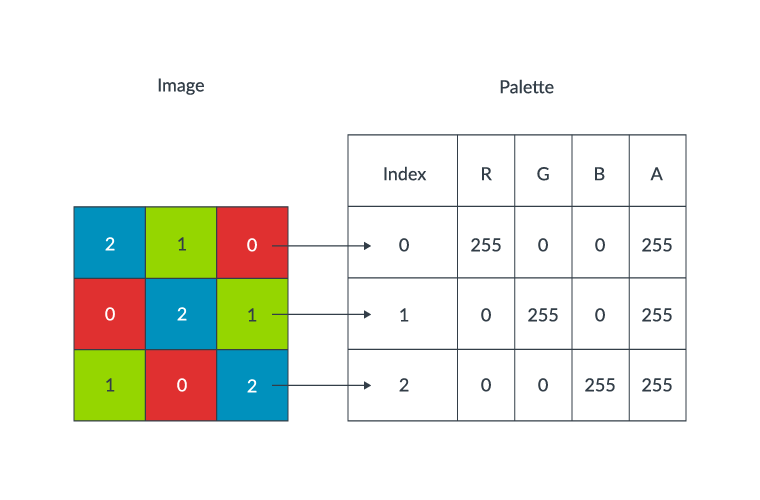### Unoptimized implementation

The original implementation of the palette expansion algorithm can be found in `png_do_expand_palette()`. The code iterates over every pixel, looking up each palette index (`*sp`) and adding the corresponding RGBA values to the output stream.

```for (i = 0; i < row_width; i++)
{
if ((int)(*sp) >= num_trans)
*dp-- = 0xff;
else
*dp-- = trans_alpha[*sp];
*dp-- = palette[*sp].blue;
*dp-- = palette[*sp].green;
*dp-- = palette[*sp].red;
sp--;
}```

### Neon-optimized implementation

The optimized code uses Neon instructions to parallelize the data transfer and restructuring. The original code individually copied across each of the RGBA values from the index. The optimized code uses Neon intrinsics to construct a four-lane vector containing the R, G, B, and A values. This vector is then stored into memory. The optimized code using Neon intrinsics is as follows:

```for(i = 0; i + 3 < row_width; i += 4) {
uint32x4_t cur;
png_bytep sp = *ssp - i, dp = *ddp - (i << 2);
cur = vld1q_dup_u32 (riffled_palette + *(sp - 3));
cur = vld1q_lane_u32(riffled_palette + *(sp - 2), cur, 1);
cur = vld1q_lane_u32(riffled_palette + *(sp - 1), cur, 2);
cur = vld1q_lane_u32(riffled_palette + *(sp), cur, 3);
vst1q_u32((void *)dp, cur);
}```

Intrinsic Description
`vld1q_dup_u32` Load all lanes of a vector with the same value from memory.
`vld1q_lane_u32` Load a single lane of a vector with a value from memory.
`vst1q_u32` Store a vector into memory.

### Results

By using vectors to speed up the data transfer, performance gains in the range 10% to 30% have been observed.

This optimization started shipping in Chromium M66 and libpng version 1.6.36.

The following resources provide additional information about the `png_do_expand_palette()` optimization:

## Pre-multiplied alpha channel data

The color of each pixel in a PNG image is defined by an RGB triple. An additional value, called the alpha channel, specifies the opacity of the pixel. Each of the R, G, B, and A values are integers between 0 and 255. An alpha value of 0 means the pixel is transparent and does not appear in the final image. A value of 255 means the pixel is totally opaque and obscures any other image data in the same location.

When rendering a PNG image, the browser needs to calculate pre-multiplied alpha data. That is, the RGB data for each pixel must be multiplied by the corresponding alpha channel value. This calculation produces scaled RGB data that accounts for the opacity of the pixel.

The following diagram shows the same RGB pixel scaled by three different alpha values: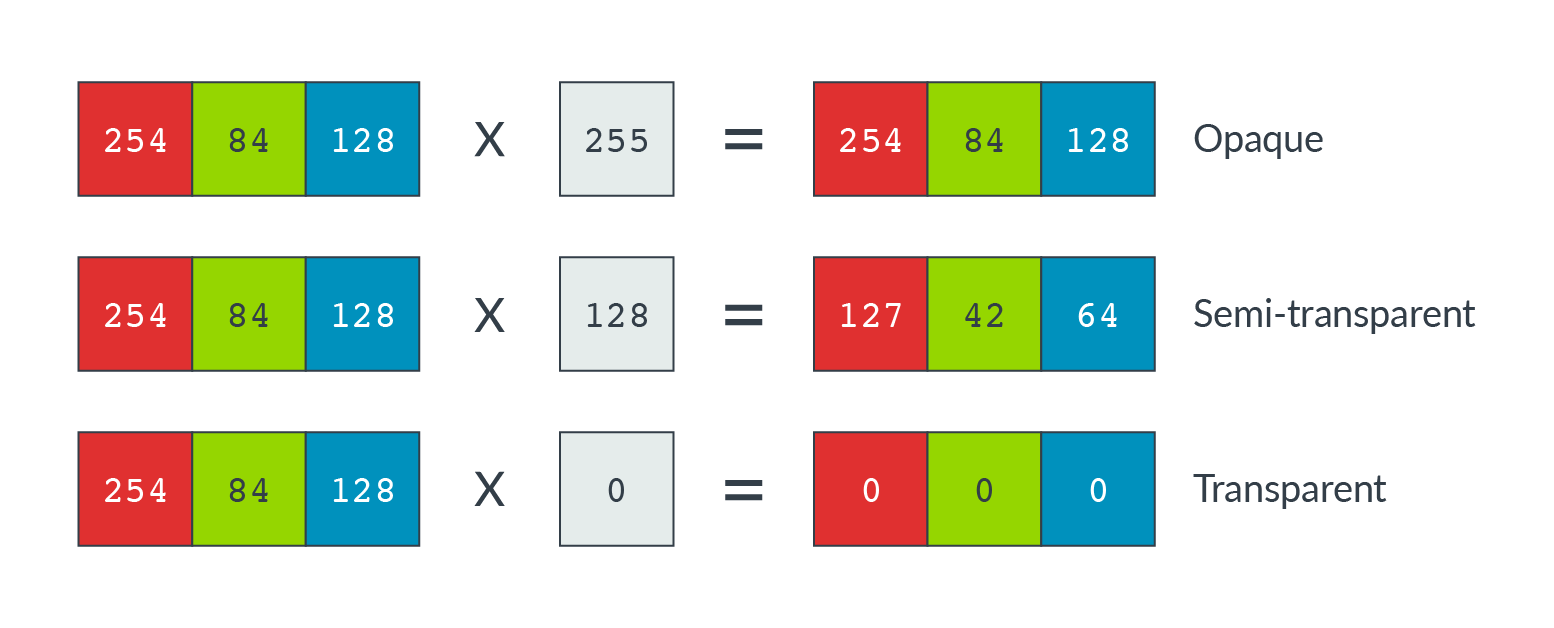Each scaled color value is calculated as you can see in the following code:

`Scaled_RGB_value = straight_rgb_value x (alpha_value / 255)`

### Unoptimized implementation

In Chromium, the code that performs this calculation is the `ImageFrame::setRGBAPremultiply()` function. Before Neon optimization, this function had the following implementation:

```static inline void setRGBAPremultiply(PixelData* dest,
unsigned r,
unsigned g,
unsigned b,
unsigned a) {
enum FractionControl { RoundFractionControl = 257 * 128 };

if (a < 255) {
unsigned alpha = a * 257;
r = (r * alpha + RoundFractionControl) >> 16;
g = (g * alpha + RoundFractionControl) >> 16;
b = (b * alpha + RoundFractionControl) >> 16;
}

*dest = SkPackARGB32NoCheck(a, r, g, b);
}```

This unoptimized function operates on a single RGBA value at a time, multiplying each of the R, G, and B values by the alpha channel.

### Neon-optimized implementation

The serial data processing performed in the unoptimized implementation provides an opportunity for Neon optimization. Rather than operating on a single data value at a time, we can:

• Load the RGBA data into separate R, G, B and A input vectors. Use a de-interleaved load. In this case, that means loading every fourth data value into the same register.
• Multiply each data lane with its corresponding alpha value simultaneously.
• Store the scaled data with an interleaved store. This means storing values from each of the four registers into adjacent memory locations, to produce an output stream of scaled RGBA data.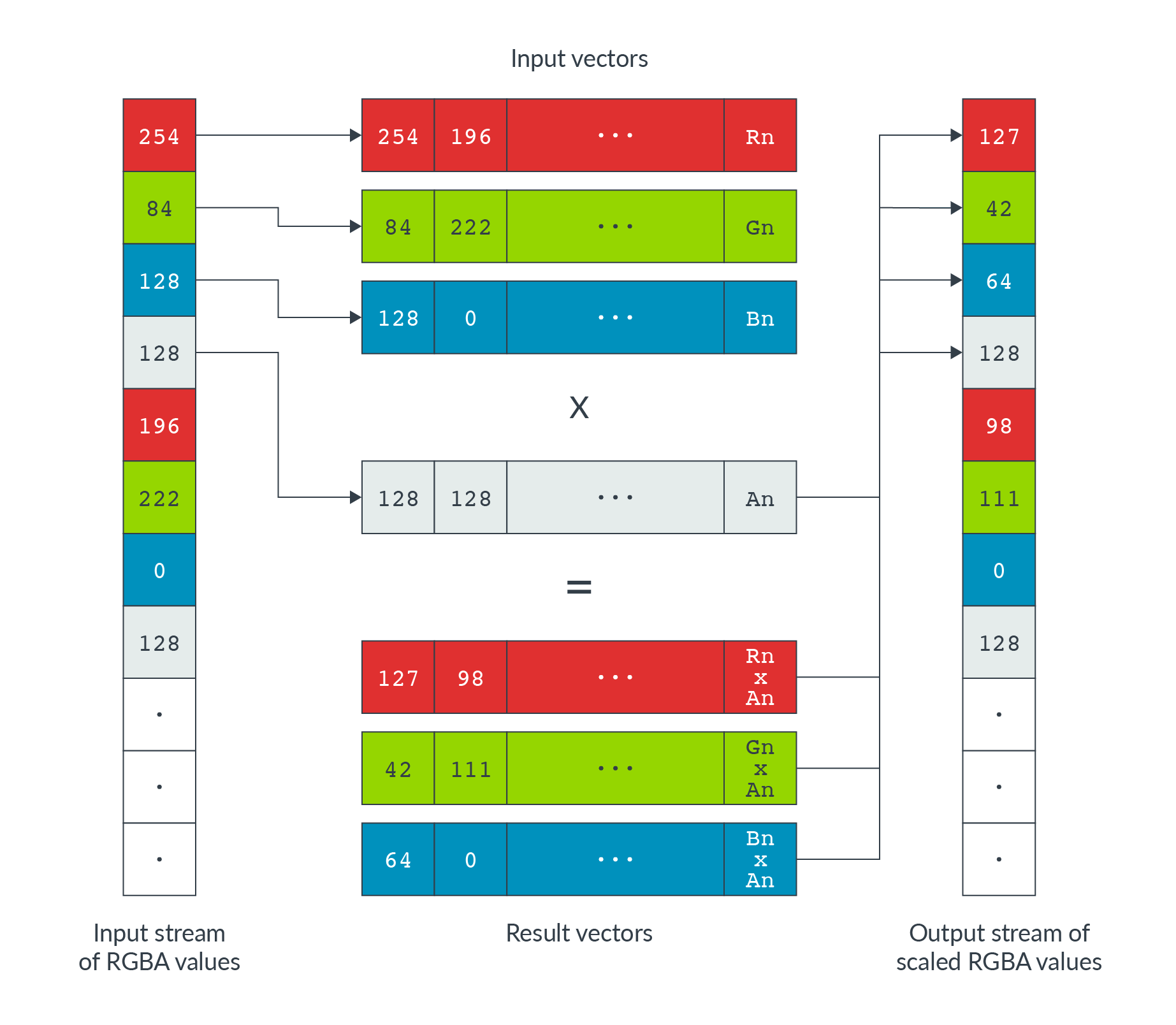The Neon optimized code is as follows:

```static inline void SetRGBAPremultiplyRowNeon(png_bytep src_ptr,
const int pixel_count,
ImageFrame::PixelData* dst_pixel,
// Input registers.
uint8x8x4_t rgba;

// Scale the color channel by alpha - the opacity coefficient.
auto premultiply = [](uint8x8_t c, uint8x8_t a) {
// First multiply the color by alpha, expanding to 16-bit (max 255*255).
uint16x8_t ca = vmull_u8(c, a);
// Now we need to round back down to 8-bit, returning (x+127)/255.
// (x+127)/255 == (x + ((x+128)>>8) + 128)>>8.  This form is well suited
// to NEON: vrshrq_n_u16(...,8) gives the inner (x+128)>>8, and
// vraddhn_u16() both the outer add-shift and our conversion back to 8-bit.
};

.
.
.

// Main loop

rgba = vld4_u8(src_ptr);

// Premultiply with alpha channel
rgba.val = premultiply(rgba.val, rgba.val);
rgba.val = premultiply(rgba.val, rgba.val);
rgba.val = premultiply(rgba.val, rgba.val);

// Write back (interleaved) results to memory.
vst4_u8(reinterpret_cast<uint8_t*>(dst_pixel), rgba);

}```

Intrinsic Description
`vmull_u8` Vector multiply.
`vraddhn_u16` Vector rounding addition.
`vrshrq_n_u16` Vector rounding shift right.
`vld4_u8` Load multiple 4-element structures to four vector registers.
`vst4_u8` Store multiple 4-element structures from four vector registers.

### Results

This optimization gave results in the region of 9% improvement.

The following resources provide additional information about the `ImageFrame::setRGBAPremultiply()` optimization:

## Next steps

This guide has shown how we identified optimization opportunities within the Chromium open-source codebase. The guide also provides detail about several specific optimizations made using Neon intrinsics.

One more notable optimization was a 20% increase in performance by optimizing `inflate_fast()` to use Neon intrinsics to perform long loads and stores in the byte array.

The result of all these optimizations was a 2.9x boost to PNG decoding performance. The following figure shows the decoding time improvement, in milliseconds, for test images comparing unoptimized zlib to Neon-optimized zlib: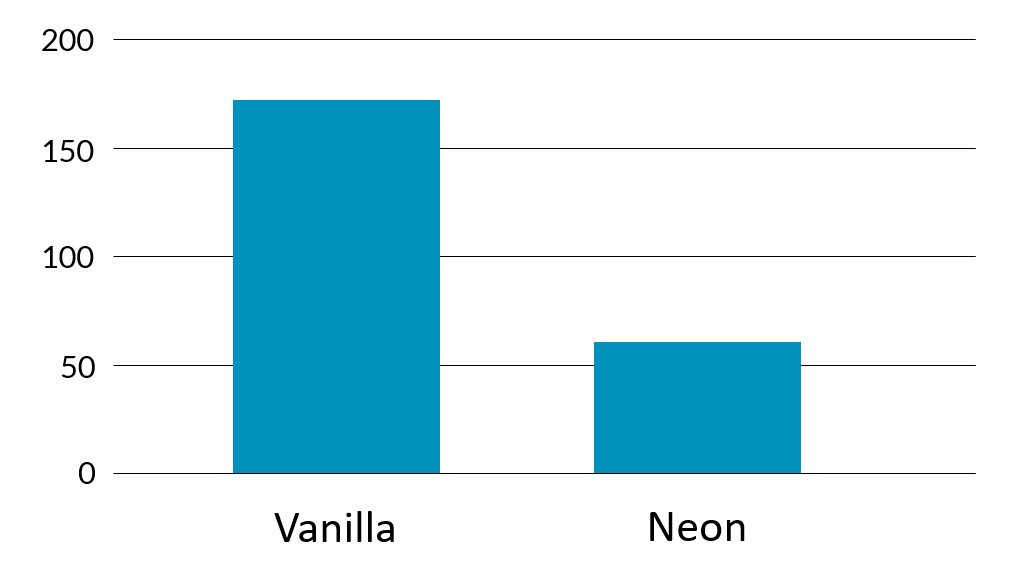Optimizations were validated using representative data sets. For PNG, we used three sets of test data:

For more information about Neon programming in general, see the Neon Programmer's Guide for Armv8-A on the Arm Developer website.